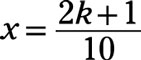##### Pre-Calculus: 1001 Practice Problems For Dummies (+ Free Online Practice)The graphs of trigonometric functions are usually easily recognizable — after you become familiar with the basic graph for each function and the possibilities for transformations of the basic graphs.

Trig functions are periodic. That is, they repeat the same function values over and over, so their graphs repeat the same curve over and over. The trick is to recognize how often this curve repeats and where one of the basic graphs starts for a particular function.

An interesting feature of four of the trig functions is that they have asymptotes — those not-really-there lines used as guides to the shape of a curve. The sine and cosine functions don’t have asymptotes, because their domains are all real numbers. The other four functions have vertical asymptotes to mark where their domains have gaps.

You’ll work with the graphs of trigonometric functions in the following ways:

• Marking any intercepts on the x-axis to help graph the curves

• Locating and drawing in vertical asymptotes for the tangent, cotangent, secant, and cosecant functions

• Computing the change in the period of a function based on some transformation

• Adjusting the amplitude of the sine or cosine when the basic curve has a multiplier

• Making sideways moves when transformations involve horizontal translations

• Moving trig functions upward or downward with vertical translations

When graphing trigonometric functions, some challenges will include

• Not misreading the period of the trig function when a transformation involves a fraction

• Drawing enough full cycles of the curve to show its characteristics properly

• Marking the axes appropriately for the situation

• Making use of intercepts when they’re helpful in a graph

## Practice problems

1. Sketch the graph of the function: f(x) = tan(4x)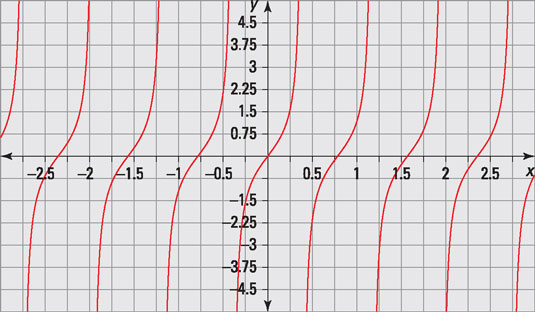Credit: Illustration by Thomson Digital

The given function is f(x) = tan(4x).

Using f(x) = AtanBx, the period of the function is determined by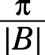therefore, the graph is the standard tangent function, except with a period of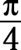2. Give a rule for the equations of the asymptotes. Then sketch the graph of the function: f(x) = 4sec(5x)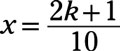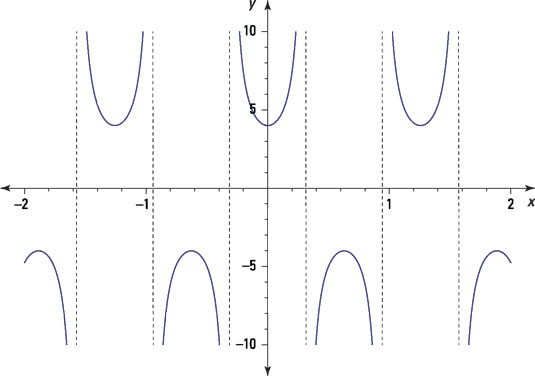Credit: Illustration by Thomson Digital

Use g(x) = AsinB(x + C) + D, where A is the amplitude,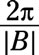is the period, C represents a horizontal shift, and D represents a vertical shift. For f(x) = 4sec(5x), the period is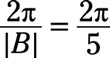The multiplier 4 brings the upper curves down to 4 and the lower curves up to ‒4.

The asymptotes are found where the reciprocal of the secant, f(x) = 4cos(5x), is equal to 0: cos5x = 0 when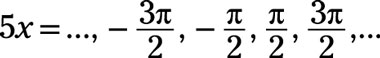Solving for x, you divide each term by 5 to get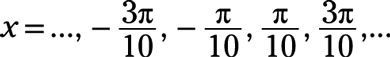Letting k be an integer, the general rule for the equations of the asymptotes is